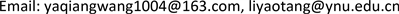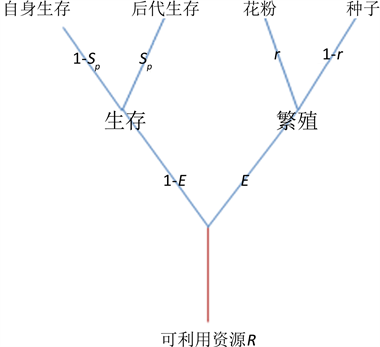﻿ 一类资源分配策略模型的推广 Generalized for a Class of Resource Allocation Strategy Model

Vol.06 No.06(2017), Article ID:22108,6 pages
10.12677/AAM.2017.66088

Generalized for a Class of Resource Allocation Strategy Model

Yaqiang Wang1, Yaotang Li2

1Institute of Mathematics and Information Science, Baoji University of Arts and Sciences, Baoji Shaanxi

2School of Mathematics and Statistics, Yunnan University, Kunming YunnanReceived: Aug. 26th, 2017; accepted: Sep. 12th, 2017; published: Sep. 19th, 2017ABSTRACT

Based on the classic resource allocation model, a general resource allocation strategy model is presented which includes reproductive allocation, survival allocation and sex allocation. From the analyzing the generalized model, we find that: 1) when the adult survivorship, the fitness of female and the juvenile survivorship are linear function of their respective resource investment, and the fitness of male is power function of its respective resource investment, reproductive allocation is independent on sex allocation; 2) when reproductive allocation is greater than 0 and smaller than 0.5, survival allocation increases as reproductive allocation increases; when reproductive allocation is greater than 0.5 and smaller than 1, survival allocation increases as reproductive allocation decreases; in particular, when reproductive is equal to 0.5, survival allocation is independent on reproductive allocation. These results provide a reasonable explanation for the phenomenon of “more reproducing but less caring” and “less reproducing but more caring” in the plant kingdom.

Keywords:Reproductive Allocation, Sex Distribution, Survival Distribution, Evolutionary Stable Strategies, Fitness

1宝鸡文理学院，数学与信息科学学院，陕西 宝鸡

2云南大学，数学与统计学院，云南 昆明1. 引言

1994年，张大勇和王刚注意到了繁殖分配和性别分配是共用一个有限资源的，因此他们将繁殖分配和性分配纳入到同一个理论框架进行研究   。通过这种整合研究揭示出了繁殖分配与性别分配独立研究所需的前提条件。然而，他们的模型忽略另一个在植物繁殖中发挥重要作用的资源分配——存活分配，存活分配是指植物将用于存活的资源中一部分资源用来维持后代存活。同时，张大勇和王刚的模型及其推广模型将后代存活率看成一个常数，这也与实际情况不符  。事实上，后代存活率在很大程度上是依赖于用于后代存活的资源比例，即存活分配。因此，将繁殖分配、存活分配和性别分配纳入到同一个框架研究是必要的。然而，在已有的文献中，笔者还没有见到将繁殖分配、存活分配和性别分配整合在同一个框架下的文献。

2. 包含繁殖分配、存活分配和性别分配的进化稳定的资源分配模型

2.1. 经典的资源分配模型回顾

1994年，张大勇和王刚在文  中提出了如下资源分配模型：

$\left\{\begin{array}{l}N\left(T+1\right)=N\left(T\right)P\left(1-E\right)+N\left(T\right)f\left[E\left(1-r\right)\right]C\\ {N}^{*}\left(T+1\right)={N}^{*}\left(T\right)\left\{P\left(1-{E}^{\prime }\right)+\frac{f\left[{E}^{\prime }\left(1-{r}^{\prime }\right)\right]C}{2}+\frac{m\left({E}^{\prime }{r}^{\prime }\right)}{2m\left(Er\right)N\left(T\right)}N\left(T\right)f\left[E\left(1-r\right)\right]C\right\}\end{array}$ ， (1)

2.2. 模型的基本假设

$P=P\left(\left(1-E\right)\left(1-{S}_{p}\right)\right)$ , $f=f\left(E\left(1-r\right)\right)$$m=m\left(Er\right)$$C=C\left(\left(1-E\right){S}_{p}\right)$ .

$N\left(T+1\right)=N\left(T\right)\left[f\left(E\left(1-r\right)\right)C\left(\left(1-E\right){S}_{p}\right)+P\left(\left(1-E\right)\left(1-{S}_{p}\right)\right)\right]$

${\lambda }_{AA}=f\left(E\left(1-r\right)\right)C\left(\left(1-E\right){S}_{p}\right)+P\left(\left(1-E\right)\left(1-{S}_{p}\right)\right)$ . (2)

$\begin{array}{c}{N}^{*}\left(T+1\right)={N}^{*}\left(T\right)\left[P\left(\left(1-{E}^{\prime }\right)\left(1-{{S}^{\prime }}_{p}\right)\right)+\frac{f\left({E}^{\prime }\left(1-{r}^{\prime }\right)\right)C\left(\left(1-{E}^{\prime }\right){{S}^{\prime }}_{p}\right)}{2}\\ \text{\hspace{0.17em}}\text{\hspace{0.17em}}+\frac{m\left({E}^{\prime }{r}^{\prime }\right)}{2N\left(T\right)m\left(Er\right)}N\left(T\right)f\left(E\left(1-r\right)\right)C\left(\left(1-{E}^{\prime }\right){{S}^{\prime }}_{p}\right)\right]，\end{array}$ (3)Figure 1. Schematic of resource allocation

${\lambda }_{AB}=P\left(\left(1-{E}^{\prime }\right)\left(1-{{S}^{\prime }}_{p}\right)\right)+\frac{C\left(\left(1-{E}^{\prime }\right){{S}^{\prime }}_{p}\right)}{2}\left[f\left({E}^{\prime }\left(1-{r}^{\prime }\right)\right)+\frac{f\left(E\left(1-r\right)\right)}{m\left(Er\right)}m\left({E}^{\prime }{r}^{\prime }\right)\right]$ .(4)

${\frac{\partial {\lambda }_{AB}}{\partial {E}^{\prime }}=0|}_{\left({E}^{\prime },{r}^{\prime },{{S}^{\prime }}_{p}\right)=\left(E,r,{S}_{p}\right)}，$ (5)

${\frac{\partial {\lambda }_{AB}}{\partial {r}^{\prime }}=0|}_{\left({E}^{\prime },{r}^{\prime },{{S}^{\prime }}_{p}\right)=\left(E,r,{S}_{p}\right)}，$ (6)

${\frac{\partial {\lambda }_{AB}}{\partial {{S}^{\prime }}_{p}}=0|}_{\left({E}^{\prime },{r}^{\prime },{{S}^{\prime }}_{p}\right)=\left(E,r,{S}_{p}\right)}.$ (7)

3. 进化稳定的资源分配策略

$P=P\left(\left(1-E\right)\left(1-{S}_{P}\right)\right)={k}_{1}\left(1-E\right)\left(1-{S}_{P}\right)$ ， (8)

$f=f\left(E\left(1-r\right)\right)={k}_{2}\left[E\left(1-r\right)\right]$ ， (9)

$C=C\left(\left(1-E\right){S}_{P}\right)={k}_{3}\left(\left(1-E\right){S}_{P}\right)$ ， (10)

$m=m\left(Er\right)={k}_{4}{\left(Er\right)}^{n}$ ， (11)

$\left\{\begin{array}{l}r=\frac{n}{n+1}\\ E=\frac{2{k}_{1}}{{k}_{2}{k}_{3}}\\ {S}_{p}=\frac{E}{1-E}\end{array}.$ (12)

4. 结果与讨论

$1/2 时，生存分配随着繁殖分配增大而减小；特别地当 $E=1/2$ 时，生存分配不随繁殖分配的变化而变化。在植物繁殖过程经常会出现少生优养和多生劣养的现象，在苹果、梨等一些主要果树上这类现象表现得更为明显  。事实上，当果树结果多时，即分配给用于繁殖的资源较多(模型中 $1/2 的情况)时，消耗的养分多，枝条内养分贫乏，即用于幼果存活的资源就变少，从而导致许多幼果不能发育而脱落，出现结果较多而果存活率较低的现象，即“多生劣养”的现象。而当果树结果较少时，即分配给用于繁殖的资源较少(模型中 $0 的情况)时，枝条内养分充分，从而出现结果较少而存活较高的现象，即“少生优养”的现象。本文的这个结果表明：许多植物可以根据外部环境来调整不同繁殖分配策略，使得繁殖分配或大或小，进而导致“多生劣养”或“少生优养”的现象。这也很好地解释了为什么有些植物会出现结果的大小年现象。

Generalized for a Class of Resource Allocation Strategy Model[J]. 应用数学进展, 2017, 06(06): 734-739. http://dx.doi.org/10.12677/AAM.2017.66088

1. 1. 赵志刚, 杜国祯, 刘左军. 雌雄同花植物的性分配[J]. 生态学报, 2005, 25(10): 2725-2733.

2. 2. 张大勇. 理论生态学研究[M]. 北京: 高等教育出版社, 2000: 66-78.

3. 3. 赵志刚, 杜国祯, 任青吉. 5种毛茛科植物个体大小依赖的繁殖分配和性分配[J]. 植物生态学报, 2004, 28(1): 9- 16.

4. 4. Charnov, E.L. (1982) The Theory of Sex Allocation. Monographs in Population Biology, 18, 121-129.

5. 5. Bell, G. (1980) The Costs of Reproduction and Their Consequences. The American Naturalist, 116, 45-76. https://doi.org/10.1086/283611

6. 6. Zhang, D.Y. and Wang, G. (1994) Evolutionarily Stable Reproductive Strategies in Sexual Organisms: An Integrated Approach to Life-History Evolution and Sex Allocation. American Naturalist, 144, 65-75. https://doi.org/10.1086/285661

7. 7. 苏晓磊, 曾波, 乔普, 阿依巧丽, 黄文军. 冬季水淹对秋华柳的开花物候及繁殖分配的影响[J]. 生态学报, 2010, 30(10): 2585-2592.

8. 8. 侯勤正, 叶广继, 马小兵, 苏雪, 张世虎, 孙坤. 青藏高原不同生境下湿生扁蕾(Gentianopsis paludosa)个体大小依赖的繁殖分配[J]. 生态学报, 2016, 36(9): 2686-2694.

9. 9. Wenk, E.H. and Falster, D.S. (2015) Quantifying and Understanding Reproductive Allocation Schedules in Plants. Ecology & Evolution, 5, 5521-5538. https://doi.org/10.1002/ece3.1802

10. 10. Baldanzi, S., Mcquaid, C.D. and Porri, F. (2015) Temperature Effects on Reproductive Allocation in the Sandhopper Talorchestia capensis. Biological Bulletin, 228, 181-191. https://doi.org/10.1086/BBLv228n3p181

11. 11. Brzyski, J.R., Taylor, W. and Mcletchie, D.N. (2014) Reproductive Allocation between the Sexes, across Natural and Novel Habitats, and Its Impact on Genetic Diversity. Evolutionary Ecology, 28, 247-261. https://doi.org/10.1007/s10682-013-9672-9

12. 12. West, S.A. (2010) Sex Allocation. Princeton: Princeton University Press, 257-273. https://doi.org/10.1038/srep33976

13. 13. Wang, Y.Q., Li, Y.T. and Wang, R.W. (2016) The Evolution of Optimal Re-source Allocation and Mating Systems in Hermaphroditic Perennial Plants. Scientific Reports, 6, 33976.

14. 14. Fudenberg, D. and Tirole, J. (1991) Game Theory. Mit Press Books, 841-846.

15. 15. 书睿. 果树的大小年现象[J]. 西北园艺: 果树专刊, 2013(3): 50-51.